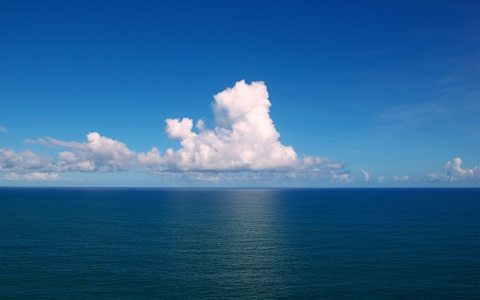WE USE COOKIES           ** FACEBOOK PAGE
This computer wallpaper features fluffy clouds floating above the Atlantic Ocean. The Atlantic Ocean is the second largest ocean on Earth, with a total area of 41,100,000 square miles (106,400,000 square km), covering about 22 percent of the Earth's surface. The only larger ocean is the Pacific. Image by Tiago Fioreze.## Download Atlantic Ocean wallpaper | Leave a Comment

### function roundNumber(b,c){var a=Math.round(b*Math.pow(10,c))/Math.pow(10,c);return a}function resPrints(){var b=screen.width;var c=screen.height;var a=roundNumber(b/c,2);var d="Your display Resolution: <strong> "+b+" x "+c+" </strong> | Aspect Ratio:<strong> ";if(a==1.25||a==1.33){d+="Standard 4:3"}else{if(a==1.6||a==1.67){d+="Wide 8:5"}else{if(a==1.78){d+="HD 16:9"}else{if(a==1.76){d+="Mobile PSP"}else{if(a==0.75||(a==1.33&&b<=640)){d+="Mobile VGA"}else{if(a==0.6||(a==1.67&&b<=800)){d+="Mobile WVGA"}else{if(a==0.67||(a==1.5&&b==480)){d+="Mobile iPhone"}else{if(a==0.8){d+="Mobile Phone"}else{d="Unknown"}}}}}}}}d+="</strong>";document.write(d)}; resPrints();

Wallpaper details:
Added: Thursday, 03 May 2012 18:43
Downloads: 45534
Sub Categories:
License: Free for personal wallpaper use only